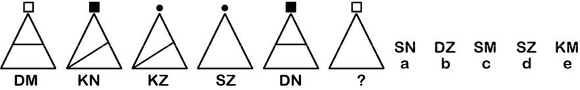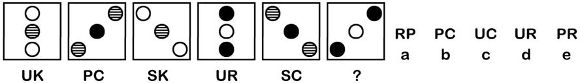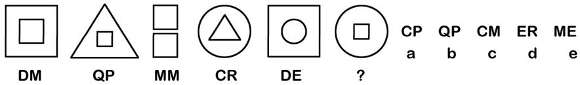Latest Results:

# 11plus Codes sample papers

Solve this Question

#### By observing the pattern of alphabetical codes mentioned below every question figure, what will be the missing code?1. a 2. b 3. c 4. d 5. e

Solve this Question

#### By observing the pattern of alphabetical codes mentioned below every question figure, what will be the missing code?1. a 2. b 3. c 4. d 5. e

#### By observing the pattern of alphabetical codes mentioned below every question figure, what will be the missing code?